In :
#%qtconsole

In :
from IPython.core.display import HTML
def css_styling():
return HTML(styles)
css_styling()

Out:
In :
import json
matplotlib.rcParams.update(s)


## Buffon's Needle¶

The problem is to randomly drop a needle on the unit square and count the number of times that the needle touches (or "cuts") the edge of the square. This is a well-studied problem, but instead of the usual tack of going directly for the analytical result (see appendix), we instead write a quick simulation that we'll later refine to obtain more precise results more efficiently. This methodology is common in Monte Carlo methods and we will work through this example in careful detail.

Buffon's needle is a classic problem that is easy to understand, and complex enough to be characteristic of much more difficult problems encountered in practice. The overall idea is that when the problem is too complex to analyze analytically (at least in the general case), we write representative computer models that can drive a numerical solution and motivate analytical solutions, even if only on special cases.

## Setting up the Simulation¶

The following code-block sets up the random needle position and orientation and creates the corresponding matplotlib primitives to be drawn in the figure. As shown, the simulation chooses a random center point for the needle in the unit square and then chooses a random angle $\theta \in (0,\pi)$ as the needle's orientation. Because the needle pivots at its center, we only need $\theta$ in this range.

In :
from __future__ import  division
from matplotlib.lines import Line2D
from matplotlib.patches import Rectangle

def needle_gen(L=0.5):
'''Drops needle on unit square. Return dictionary describing needle position and
orientation. The cut key in the dictionary is True when the needle intersects
an edge of the unit square and is False otherwise.

L := length of needle (default = 0.5)
'''
assert 0<L<1
xc,yc = rand(2) # uniform random in [0,1] for each coordinate dimension
ang = rand()*pi # uniform random angle in [0,pi]
x0,y0 = xc-L/2*cos(ang),yc-L/2*sin(ang) # coordinates for one end of needle
xe,ye = xc+L/2*cos(ang),yc+L/2*sin(ang) # coordinates for the other end
not_cut = (0<x0<1) and (0<y0<1) and (0<xe<1) and (0<ye<1)
return dict(x0=x0,y0=y0,xc=xc,yc=yc,xe=xe,ye=ye,ang=ang,cut=( not not_cut ))

def draw_needle(ax,d):
'''
Draw needle symbol on given axis.

If red, then the line cuts an edge of the unit square. If blue, then it does not.
One end of the needle is marked with a o symbol and the other end is unmarked.

ax := matplotlib axes to draw needle symbol
d := dictionary output from needle_gen
'''
for i,j in d.iteritems(): exec('%s=%r'%(i,j)) # dump dictionary in local namespace
if cut:
line= [Line2D([x0,xe],[y0,ye],color='r',alpha=0.3),
Line2D([xc],[yc],marker='o',color='r')]
else:
line= [Line2D([x0,xe],[y0,ye],alpha=0.3),
Line2D([xc],[yc],marker='o',alpha=0.3)]
for i in line:


Now, we can simulate the dropping event on the square (yellow background ). The red needles cut the edge of the square and the blue ones are completely contained therein. The circle marker shows the needle position.

In :
L=0.5

samples = [needle_gen() for i in range(500)]

def draw_sim(samples,L=0.5):
'Draw simulation results. Package this plot for reuse later'
fig,ax=subplots()
fig.set_size_inches(5,5)

red_count=0
n=len(samples)
for k in samples:
red_count+=k['cut']
draw_needle(ax,k)

ax.set_aspect(1) # set aspect ratio to 1
ax.add_patch(Rectangle((0,0),1,1,alpha=0.3,color='y')) # unit square background is light yellow
ax.set_xlabel('x-direction')
ax.set_ylabel('y-direction')
ax.axis([-.1,1.1,-.1,1.1]) # add some space around the unit square
ax.set_title(r'$\mathbb{P}$ (cut)=%3.2f'% (red_count/n))
ax.grid()

draw_sim(samples)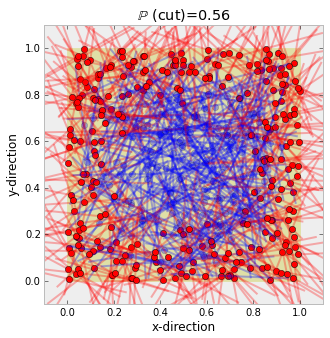The figure above is a good visual check of our simulation and helps motivate our reasoning. Now, we want to concentrate on counting the number of cuts and then use that to estimate the probability of a cut.

In :
fig,axs = subplots(1,2)
fig.set_size_inches((12,2))
ax=axs
samples=np.array([[needle_gen(L)['cut'] for i in range(300)] for k in range(100)])

ax.plot(np.mean(samples,axis=1),'o-',alpha=0.3)
ax.axis(xmax=samples.shape)
ax.set_xlabel(r'$trial$',fontsize=18)
ax.set_ylabel('$\mathbb{\hat{P}}(cut)$',fontsize=18)
ax.set_title('$\overline{\mathbb{\hat{P}}}(cut) = %3.3f,\hat{\sigma}=%3.3f$'%
(samples.mean(),(samples.mean(1)).std()))
ax.grid()

ax = axs
ax.hist( samples.mean(1));
ax.set_title('Distribution of Mean Estimates');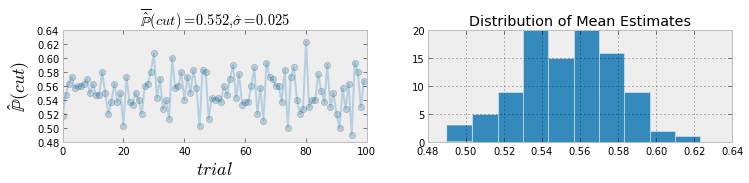The figure above shows some statistical history of our Monte Carlo simulation. The samples array in the prior code-block is a 100x300 matrix of True/False values indicating cut or no-cut. The overall average of this array is our estimate of the probability of a cut. In other words, we count the number of cuts and divide by the total number of needle drops to obtain the estimated probability (approximately 55% in this case of $L=1/2$). The plot on the left shows the average across each of the 300 columns. Thus, there are 100 such column-wise averages (recall the samples matrix is 100x300). The title shows the estimated standard deviation across these 100 columnwise averages.

The plot on the right shows a histogram of the column-wise averages. Why did we partition the simulated samples like this and compute column-wise instead of just using all the data at once? Because we want to get a sense of the spread of the estimates by computing the standard error. If we used all our samples at once, we would not be able to do this even though we'll still use our average of these averages to get our ultimate probability of a cut!

Naturally, if we wanted to improve the standard deviation of our ensemble of columnwise means, we could simply run a larger simulation, but remember that the standard error only improves at the rate of $\sqrt{N}$ (where $N$ is the number of samples) so we'd need a sample run four times as large to improve the standard error by a factor of two. Furthermore, we need to understand the mechanics of this problem better per unit of computing time.

## Relative Errors and Using Weighting for Better Efficiency¶

There's nothing wrong with the way we have been using the simulation so far, but in the first figure all of those blue needles did not help us compute the probability we were after because they did not touch the edges of the square. Yes, we could reverse the reasoning and use the blue instead of the red for our estimation, but that does not escape the basic problem. For example, if the length of the needle were really short in comparison, then there would have been a lot of blue in our initial plot (try this using this IPython notebook!). In the extreme case, we could find ourselves in a situation where we run the simulation for a long time and not have a single cut! We want to use our valuable computer time to create samples that will improve our estimated solution.

To recap, we computed a matrix of (100x300) cases of needle drops. Each of element of samples in the code above is a True/False value as to whether or not the needle touched the edge of the square. We averaged over the 300 columns to compute the estimated probability of a cut (the mean value of the boolean array in this case). The estimated standard deviation is taken over the ensemble of these estimated means.

A common way to evaluate the quality of the simulated result is to compute the relative error, which is the ratio of the estimated standard deviation of the mean to the estimated mean using all of the samples ($R_e$). This is computed in the title of the figure below.

In :
fig,ax=subplots()
fig.set_size_inches(12,2)
ax.plot(samples.mean(1))
ax.set_title(r'$\hat{\sigma}=%2.3f,R_e=%2.3f$'% (samples.mean(1).std(),samples.mean(1).std()/samples.mean()), fontsize=18)
ax.set_xlabel('sample history index',fontsize=16)
ax.set_ylabel('$\mathbb{\hat{P}}(cut)$',fontsize=18);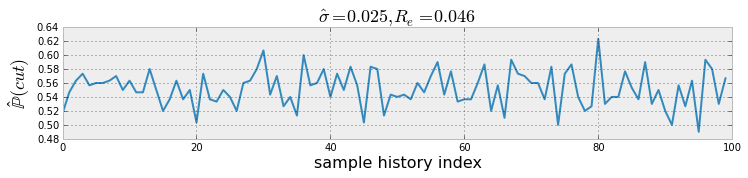So far, our relative error is pretty good (the rule of thumb is below 5%), but let's see how we do when the needle length is smaller? The code-block below draws the corresponding figure for the simulation for this case.

In :
L=0.1

samples_d=[needle_gen(L) for i in range(300*100)]
samples = np.array([k['cut'] for k in samples_d]).reshape(100,300)

def draw_sample_history(samples):
fig,ax=subplots()
fig.set_size_inches(12,2)
mn = samples.mean(1)
ax.plot(mn)
ax.set_title(r'$\hat{\sigma}=%2.3f,R_e=%2.3f$'% (mn.std(),
mn.std()/mn.mean()), fontsize=18)
ax.set_xlabel('sample history index',fontsize=16)
ax.set_ylabel('$\mathbb{\hat{P}}(cut)$',fontsize=18);

draw_sample_history(samples)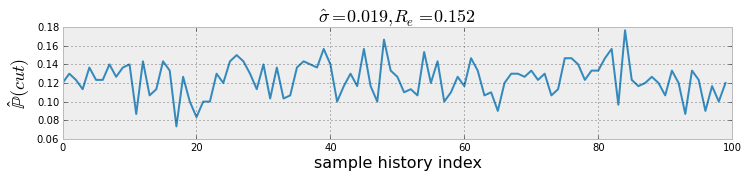The figure above shows that for shorter needles, we did not do so well for relative error. This situation is illustrated in the figure below.

In :
draw_sim(samples_d[:500])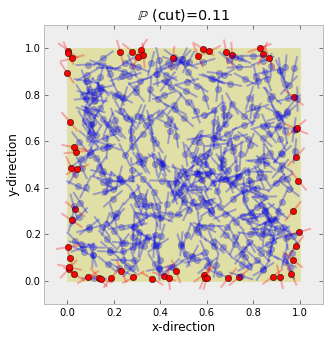The figure shows there are very few cuts in our simulation with the short needle (red needles). To remedy this, we can alter the code to bias for randomly generating x-coordinates that are within needle length of an edge instead of uniformly random in the unit interval. The code-block below makes this change for the x-coordinate.

In :
def needle_gen_b(L=0.5):
'''Drops needle on unit square using a biased strategy. Return dictionary describing
needle position and orientation. The cut key in the dictionary is True when the
needle intersects an edge of the unit square and is False otherwise.

L := length of needle (default = 0.5)
'''
assert 0<L<1
yc = rand() # uniform on unit interval
r = L*rand()/2.
xc = r if rand()<0.5 else (1-r) # pick either edge 50/50
ang = rand()*pi # uniform random angle in [0,pi]
x0,y0 = xc-L/2*cos(ang),yc-L/2*sin(ang) # coordinates for one end of needle
xe,ye = xc+L/2*cos(ang),yc+L/2*sin(ang) # coordinates for the other end
not_cut = (0<x0<1) and (0<y0<1) and (0<xe<1) and (0<ye<1)
return dict(x0=x0,y0=y0,xc=xc,yc=yc,xe=xe,ye=ye,ang=ang,cut=( not not_cut ))


Now, we re-run this simulation using this biased method.

In :
samples_b=[needle_gen_b(L) for i in range(300*100) ] # create biased samples
draw_sim(samples_b[:500])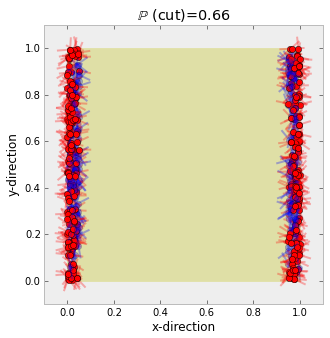The figure above shows that the samples are now clustered at both vertical edges, and, compared with the unbiased simulation, there are more red needles, indicating that we now have more relevent samples.

The next figure shows the sample history and relative error which is now much better than before.

In :
no_bias_samples = samples # save for later
samples = np.array([k['cut'] for k in samples_b]).reshape(100,300)

draw_sample_history(samples);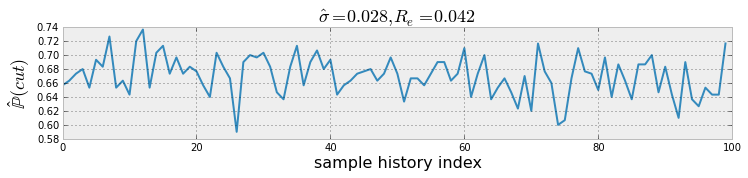Now, that we have a tighter result, we need to make sure we have not changed the result by correcting for the bias we introduced. The probability of being near the edges in the x-direction is $2 L$. We have to multiply our estimated probability by this weighting factor.

In :
fig,ax=subplots()
fig.set_size_inches(10,3)
ax.hist(no_bias_samples.mean(1),alpha=0.3,normed=1,label='unbiased')
ax.hist(samples.mean(1)*2*L,alpha=0.3,normed=1,label='biased')
ax.legend(loc=0)
ax.set_title('Biased samples more efficiently produce useful samples')

Out:
<matplotlib.text.Text at 0x82697f0>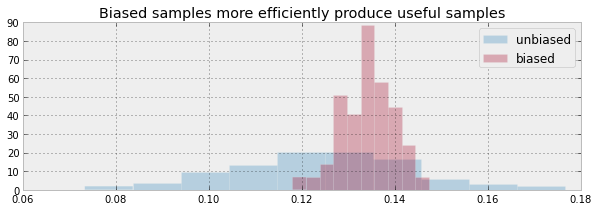The histogram above shows the spread of the biased samples is much tighter than before, which is what the relative error we just computed is getting at. Still, it's good to back this up with a quick plot.

## Summary¶

In this section, we illustrated some basic concepts of Monte Carlo methods by examing the famous problem of Buffon's needle. We showed how to code up a quick simulation that visually reveals the key issues and then how to boil this down into a simulation to compute the estimated solution. We also showed how to create biased samples to improve the overall efficiency and precision of our result.

I carefully used the word precision as opposed to accuracy because we have created a tighter estimate (as measured by relative error), but how do we know that this is the correct (i.e. accurate) answer? We don't! In this case, we can solve for the analytical solution (shown in the appendix), but in general, this is way too hard to do, otherwise we wouldn't bother with the simulation in the first place.

The reality is that we have to do what we can using Monte Carlo methods and then carefully consider their computed results using as much domain knowledge as we can muster. In this section, we made the extra effort to generate some visual results along the way as a sanity-check and doing so is always worth the extra effort, when feasible. Furthermore, this kind of intermediate result can sometimes suggest a narrow special case for which we can generate a related analytical solution that we can use to further develop our simulation.

As usual, the IPython Notebook corresponding to this section is available for download here. Play around with the various parameters as suggested above on your own to see what else you can discover about this famouse problem.

## Appendix: Analytical Results¶

In this appendix, we carefully derive the analytical solution for our problem using excessive detail. Note that $x_c$ is the needle's center. The needle is entirely contained in the square (at least in the x-direction) when the following two conditions hold:

$$0 < x_c - L/2 \cos(\theta) < 1$$$$0 < x_c + L/2 \cos(\theta) < 1$$

combining these two into one big inequality gives:

$$\max(-L/2 \cos(\theta) , L/2 \cos(\theta) ) < x_c < \min(1-L/2 \cos(\theta) , 1+L/2 \cos(\theta) )$$

In the case where $0< \theta < \pi/2$, we additionally have the following:

$$L/2 \cos(\theta) < x_c < 1-L/2 \cos(\theta)$$

Because $\theta$ is uniformly distributed between $0$ and $\pi$, for this domain of $\theta$ and $x_c$, we have the probability of the needle being contained completely in the square (in the x-direction) as the following:

$$\frac{1}{\pi}\int_0^{\pi/2} \int_{L/2 \cos(\theta)}^{1-L/2 \cos(\theta)} dx_c d\theta = \frac{1}{\pi}\int_0^{\pi/2} 1-L \cos(\theta) d\theta = \frac{1}{2} - \frac{L}{\pi}$$

Now, we can consider the other half of the domain of $\theta$, $\pi/2 < \theta < \pi$, where we have

$$-L/2 \cos(\theta) < x_c < 1+L/2 \cos(\theta)$$

Then, following the same reasoning as before we obtain:

$$\frac{1}{\pi}\int_{\pi/2}^{\pi} \int_{-L/2 \cos(\theta)}^{1+L/2 \cos(\theta)} dx_c d\theta = \frac{1}{\pi}\int_{\pi/2}^{\pi} 1+L \cos(\theta) d\theta = \frac{1}{2} - \frac{L}{\pi}$$

Thus, combining these two results gives the probability of the needle not cutting the edge in the x-direction as the following:

$$\mathbb{P}(\text{no x-cut}) = 1 - \frac{2 L}{\pi}$$

Now, we can pursue exactly the same line of reasoning for the y-direction, or, just recognize that by symmetry it would be exactly the same as for the x-direction. Nonetheless, let's be thorough and reproduce the reasoning for the y-direction, just to be on the safe side.

As before, the condition in the y-direction for the needle being entirely contained in the square in the y-direction is the following:

$$\max(-L/2 \sin(\theta) , L/2 \sin(\theta) ) < y_c < \min(1-L/2 \sin(\theta) , 1+L/2 \sin(\theta) )$$

which can be combined into one big inequality, given the restrictions on $\theta \in (0,\pi)$ as shown:

$$L/2 \sin(\theta) < y_c < 1-L/2 \sin(\theta)$$

Then, integrating this out as before, we obtain

$$\frac{1}{\pi}\int_0^{\pi} \int_{L/2 \sin(\theta)}^{1-L/2 \sin(\theta)} dy_c d\theta = \frac{1}{\pi}\int_0^{\pi} 1-L \sin(\theta) d\theta = 1 - \frac{2 L}{\pi}$$

$$\mathbb{P}(\text{no y-cut}) = 1 - \frac{2 L}{\pi}$$

which is exactly what we got for the x-direction. It's usually worth the extra work to check things like this instead of trying to be too clever too early.

Then, combining the x-direction and y-direction results, we obtain,

$$\mathbb{P}(\text{no cut}) = \left(1 - \frac{2 L}{\pi}\right)^2$$

so that

$$\mathbb{P}(\text{cut}) =1-\mathbb{P}(\text{no cut}) = 1-\left(1 - \frac{2 L}{\pi}\right)^2$$

which we code next.

In :
prob_cut= lambda l:(1-(1-2*l/pi)**2)


We can use this result later to check or Monte Carlo estimates. Try it!